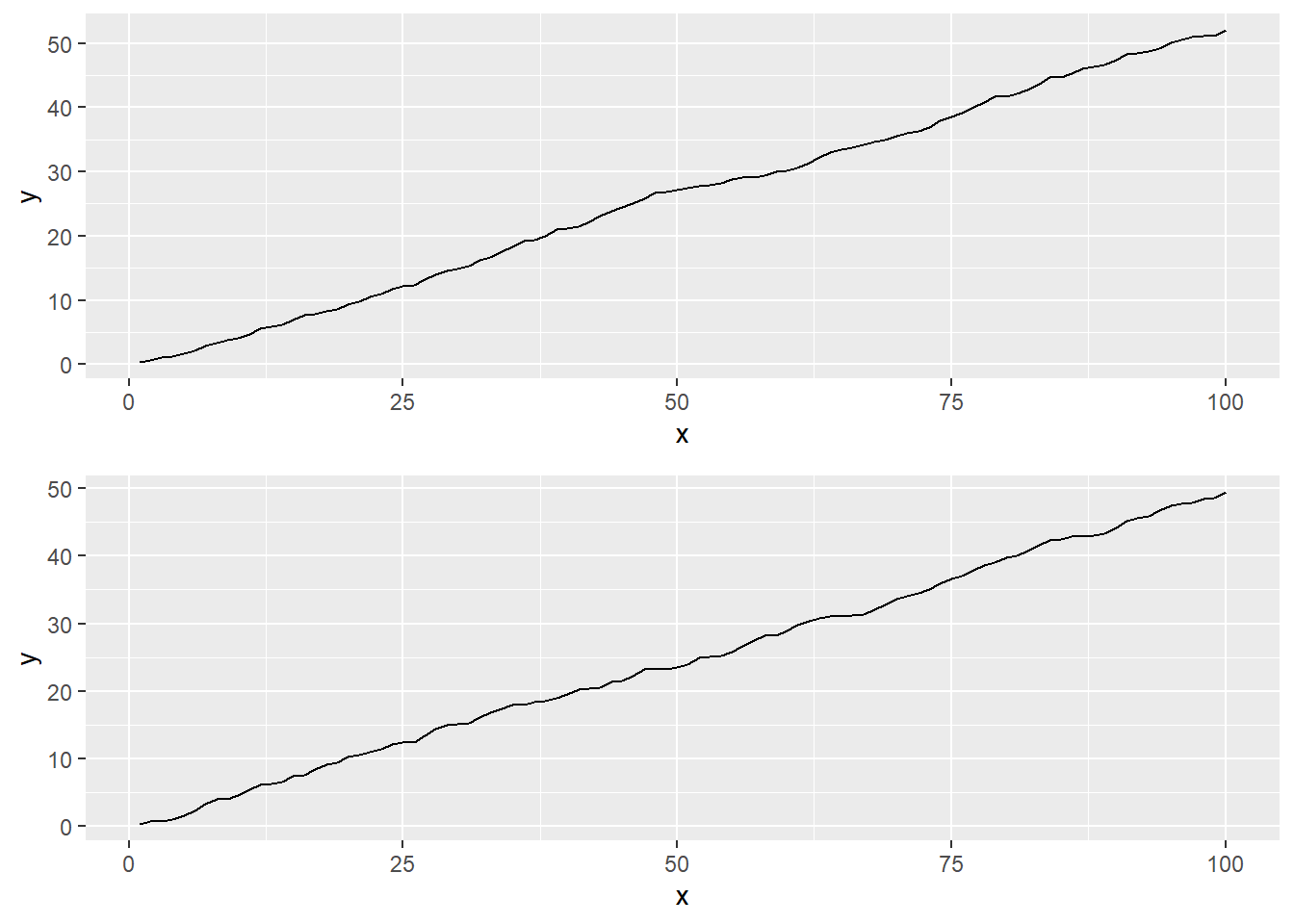# Double dispatch of S3 method

R internal
Author

Hiroaki Yutani

Published

September 17, 2018

When I tried to define an S3 class that contains multiple ggplot objects, I’ve faced the lessor-know mechanism of S3 method dispatch, double dispatch.

## Problem

Take a look at this example. `manyplot` class contains many plots, and displays them nicely when printted.

``````library(ggplot2)

set.seed(100)
d1 <- data.frame(x = 1:100, y = cumsum(runif(100)))
d2 <- data.frame(x = 1:100, y = cumsum(runif(100)))

plot_all <- function(...) {
l <- lapply(list(...), function(d) ggplot(d, aes(x, y)) + geom_line())
l <- unname(l)
class(l) <- "manyplot"
l
}

print.manyplot <- function(x, ...) {
do.call(gridExtra::grid.arrange, x)
}

p <- plot_all(d1, d2)
p``````So far, so good.

Next, I want to define `+` method, so that I can customize the plots just as I do with usual ggplot2.

```````+.manyplot` <- function(e1, e2) {
l <- lapply(e1, function(x) x + e2)
class(l) <- "manyplot"
l
}``````

But, this won’t work…

``p + theme_bw()``
``Warning: Incompatible methods ("+.manyplot", "+.gg") for "+"``
``Error in p + theme_bw(): non-numeric argument to binary operator``

What’s this cryptic error? To understand what happened, we need to dive into the concept of S3’s “double dispatch

## Double dispatch?

Usually, S3’s method dispatch depends only on the type of first argument. But, in cases of some infix operators like `+` and `*`, it uses both of their arguments; this is called double dispatch.

Why is this needed? According to Advanced R:

This is necessary to preserve the commutative property of many operators, i.e. `a + b` should equal `b + a`.

To ensure this, if both `a` and `b` are S3 objects, the method chosen in `a + b` can be (c.f. how `do_arith()` works with S3 objects):

Does `a` have an S3 method? Does `b` have an S3 method? Are the methods same? Whet method is chosen?
yes yes yes `a`’s method or `b`’s method (they are the same)
yes yes no internal method
yes no - `a`’s method
no yes - `b`’s method
no no - internal method

Here’s examples to show them clearly:

``````foo <- function(x) structure(x, class = "foo")
`+.foo` <- function(e1, e2) message("foo!")

bar <- function(x) structure(x, class = "bar")
`+.bar` <- function(e1, e2) message("bar?")

# both have the same S3 method
foo(1) + foo(1)``````
``foo!``
``NULL``
``````# both have different S3 methods
foo(1) + bar(1)``````
``Warning: Incompatible methods ("+.foo", "+.bar") for "+"``
`````` 2
attr(,"class")
 "foo"``````
``````# `a` has a method, and `b` doesn't
foo() + 1``````
``Error in structure(x, class = "foo"): argument "x" is missing, with no default``
``````# `b` has a method, and `a` doesn't
1 + foo()``````
``Error in structure(x, class = "foo"): argument "x" is missing, with no default``
``````# both don't have methods
rm(`+.foo`)
foo(1) + foo(1)``````
`````` 2
attr(,"class")
 "foo"``````

## Explanation

So, now it’s clear to our eyes what happened in the code below; they have different methods (`+.manyplot` and `+.gg`) so it falled back to internal method. But, because fundamentally they are `list`, the internal mechanism refused to add these two objects…

``p + theme_bw()``

## How can I overcome this?

Hadley says ggplot2 might eventually end up using the double-dispatch approach in vctrs. So, we can wait for the last hope.

If you cannot wait, use S4. S4 can naturally do double dispatch because their method dispatch depends on the whole combination of types of the arguments.